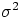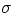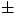Next: 10.8 Smoothing and Filtering Up: 10 MORE WAYS TO Previous: 10.6 Continuum Normalization

10.7 More Fitting Routines

You may remove a baseline from your spectrum and fit Gaussian profiles to your features with GAUSSFITS:

GAUSSFITS,W,F,NDEG,NCOMP,A,Y,SIG,comment,/prnt

 W,F Wavelengths and fluxes for your spectrum. NDEG Degree of polynomial to fit to the baseline (see BASEREM.) NCOMP Number of Gaussian components to fit. A This output parameter will contain the center positions, 1 sigma widths, and heights of each gaussian fit, and also the coefficients of the polynomial fit to the baseline. Y Fitted function. SIG 1 sigma errors in A. comment This allows you to put a comment at the top of the output plot. prnt If this keyword is set, you will be offered a chance to send the final plot to the printer.

You will be asked to mark the left and right sides of the total feature region (encompassing all gaussian components to be fit). The data outside the feature region will be used to fit the baseline, which is subtracted out. Then, you must place the crosshairs on each component peak (NCOMP of them). This procedure calls WPOLYFIT and another fitting routine called WFIT. Please see the warnings about WPOLYFIT, which apply also to WFIT.

You may also use BASEREM (called by GAUSSFITS) separately to remove a baseline from a spectrum:

BASEREM,W,F,NDEG,XL,XR,A,YBF,CHIS

 W,F Wavelengths and fluxes for spectrum from which to remove baseline. NDEG Degree of polynomial with which to fit the baseline. WL Wavelength of the left edge of the feature. WR Wavelength of the right edge of the feature. If both WL and WR are zero, then you will be allowed to select them with crosshairs. A Vector containing the coefficients of the polynomial used to approximate the baseline. YBF The calculated polynomial fit to the baseline. chis Variance of the fit.

You may also use other fitting routines to approximate the continuum (see below). It is even possible to use POINT) to select points representative of the continuum and then fit those values with one of the fitting routines below. Once a fit is calculated, it can be subtracted or divided out.

LINFIT is similar to Bevington's LINFIT, and uses a least squares fit to data with a straight line:

LINFIT,W,F,WT,A,B,st

 W,F Wavelengths and fluxes of spectrum to fit. WT Weights for spectrum to fit (can be set to W × 0+1. for uniform weighting). A Intercept of fitted line. B Slope of fitted line. st Optional statistical information about the fit, in the order in which they appear: Correlation coefficient (1=perfect fit, 0=no correlation at all) 1 sigma error of intercept 1 sigma error of slope mean value of input flux array scatter about mean (only if uniform weighting is used) scatter about fit (only if uniform weighting is used)

WPOLYFIT fits a weighted polynomial to data points using the least squares method:

WPOLYFIT,W,F,WT,NDEG,A,YFIT,CHISQR

 W,F Wavelengths and fluxes for spectrum to be fit. WT Weights for spectrum to fit (can be set to W0+1. for uniform weighting). NDEG Degree of polynomial to fit. A Coefficients of fitted polynomial. YFIT Fitted polynomial. CHISQR Reduced chi square statistic for fit.

WFIT is a general program to fit data to arbitrary shapes. It uses techniques based on Bevington's CURFIT program to linearize the fitting function.

WFIT,W,F,WT,IFIT,DELTAA,A,YFIT,SIGMA,proc=proc, \$
chisq=chisq,matherr=matherr

 W Your wavelengths or other independent variable. F Your fluxes or other dependent variable. WT Data weights. Examples: even weighting: wt=w*0.+1. instrumental errors: wt=1/(Note: neither the NEWSIPSvector nor the errors from Gex are instrumental errors.) statistical errors: wt=1/f2 IFIT Vector with the same number of elements as A (the fit parameters) containing zeroes for variable parameters and ones for fixed parameters. DELTAA Vector corresponding to A containing the increments for A (none may be zero). A Starting fit parameters appropriate to the function to fit. It will be updated with the final fit parameters. YFIT The fitted curve. SIGMA Vector of 1errors corresponding to A. proc Name of fitting algorithm. The default algorithm is called YFIT. The algorithm must have the same calling sequence as YFIT (i.e., yfit,x,a,y where x is the independent variable, a contains the fit parameters, and y is the fitted function). Example: proc='pfit'. chisqr Final value of chi-squared calculated by WFIT. matherr Results of the CHECK_MATH function executed at the end of WFIT.

The functions available for WFIT, with descriptions of their A vectors, include:

YFIT Gaussian fit.

A(3i+0) Center of ith gaussian.
A(3i+1) Width of ith gaussian.
A(3i+2) Height of ith gaussian.

MFIT Gaussian fit, with fixed widths for all gaussian components:

A(0) Width for all gaussian components.
A(2i+1) Center of ith gaussian.
A(2i+2) Height of ith gaussian.

PFIT Power law fit:  M × (x/x0)P

A(3i+0) X0
A(3i+1) N
A(3i+2) P

LORFIT Lorentzian fit:

A(3i+0) Center of ith Lorentzian
A(3i+1) FWHM of ith Lorentzian
A(3i+2) Height factor for ith Lorentzian

Caution: The programs WFIT and WPOLYFIT  may have problems with arithmetic overflow/underflow. This occurs when the dependent data are very small numerically, such as very weak IUE spectra (particularly during calculation of theparameter), and also when the slope is very flat. The program may abort under these conditions. Users can avoid this problem by scaling the data, or possibly by working with the data before applying the absolute calibration.

Another more insidious problem with these routines is that the y-intercept may be in error when the independent data are far from zero, as is usual with IUE data. This corrupts the other coefficients. Attempts to overplot the fit on the data will be unsuccessful due to the fit being off scale, i.e., the fit will simply not appear on the plot at all. Subtracting the value of the smallest wavelength from the wavelength vector prior to fitting should give reasonable results for polynomial fits e.g., wave=wave-wave(0).

These routines are called by BASEREM and by GAUSSFITS. Therefore, the problems described above can occur with them as well.Next: 10.8 Smoothing and Filtering Up: 10 MORE WAYS TO Previous: 10.6 Continuum Normalization

2/9/1998